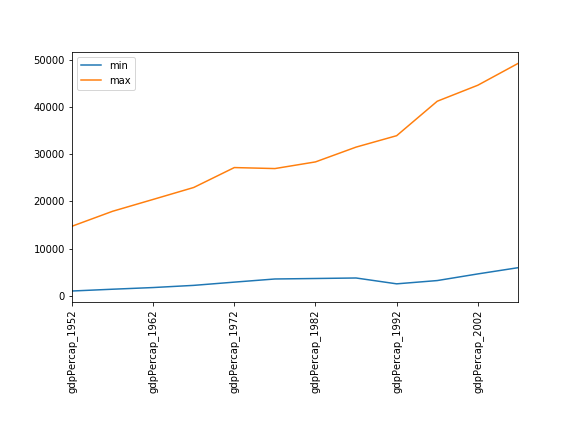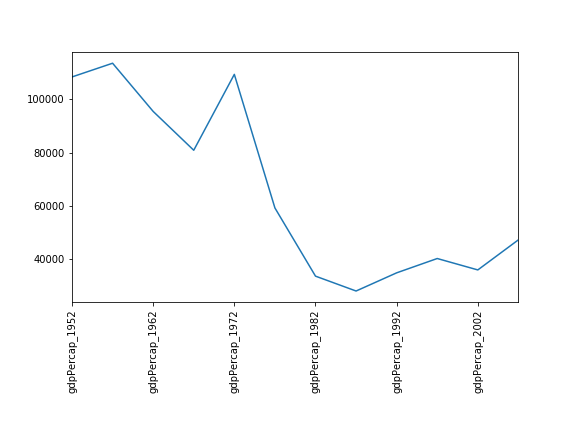# Plotting

## Overview

Teaching: 15 min
Exercises: 15 min
Questions
• How can I plot my data?

• How can I save my plot for publishing?

Objectives
• Create a time series plot showing a single data set.

• Create a scatter plot showing relationship between two data sets.

## `matplotlib` is the most widely used scientific plotting library in Python.

``````import matplotlib.pyplot as plt
``````
• Simple plots are then (fairly) simple to create.
``````time = [0, 1, 2, 3]
position = [0, 100, 200, 300]

plt.plot(time, position)
plt.xlabel('Time (hr)')
plt.ylabel('Position (km)')
``````## Display All Open Figures

In our Jupyter Notebook example, running the cell should generate the figure directly below the code. The figure is also included in the Notebook document for future viewing. However, other Python environments like an interactive Python session started from a terminal or a Python script executed via the command line require an additional command to display the figure.

Instruct `matplotlib` to show a figure:

``````plt.show()
``````

This command can also be used within a Notebook - for instance, to display multiple figures if several are created by a single cell.

## Plot data directly from a `Pandas dataframe`.

• We can also plot Pandas dataframes.
• This implicitly uses `matplotlib.pyplot`.
• Before plotting, we convert the column headings from a `string` to `integer` data type, since they represent numerical values
``````import pandas as pd

data = pd.read_csv('data/gapminder_gdp_oceania.csv', index_col='country')

# Extract year from last 4 characters of each column name
# The current column names are structured as 'gdpPercap_(year)',
# so we want to keep the (year) part only for clarity when plotting GDP vs. years
# To do this we use strip(), which removes from the string the characters stated in the argument
# This method works on strings, so we call str before strip()

years = data.columns.str.strip('gdpPercap_')

# Convert year values to integers, saving results back to dataframe

data.columns = years.astype(int)

data.loc['Australia'].plot()
``````## Select and transform data, then plot it.

``````data.T.plot()
plt.ylabel('GDP per capita')
``````## Many styles of plot are available.

• For example, do a bar plot using a fancier style.
``````plt.style.use('ggplot')
data.T.plot(kind='bar')
plt.ylabel('GDP per capita')
``````## Data can also be plotted by calling the `matplotlib``plot` function directly.

• The command is `plt.plot(x, y)`
• The color and format of markers can also be specified as an additional optional argument e.g., `b-` is a blue line, `g--` is a green dashed line.

## Get Australia data from dataframe

``````years = data.columns
gdp_australia = data.loc['Australia']

plt.plot(years, gdp_australia, 'g--')
``````## Can plot many sets of data together.

``````# Select two countries' worth of data.
gdp_australia = data.loc['Australia']
gdp_nz = data.loc['New Zealand']

# Plot with differently-colored markers.
plt.plot(years, gdp_australia, 'b-', label='Australia')
plt.plot(years, gdp_nz, 'g-', label='New Zealand')

# Create legend.
plt.legend(loc='upper left')
plt.xlabel('Year')
plt.ylabel('GDP per capita (\$)')
``````

## Adding a Legend

Often when plotting multiple datasets on the same figure it is desirable to have a legend describing the data.

This can be done in `matplotlib` in two stages:

• Provide a label for each dataset in the figure:
``````plt.plot(years, gdp_australia, label='Australia')
plt.plot(years, gdp_nz, label='New Zealand')
``````
• Instruct `matplotlib` to create the legend.
``````plt.legend()
``````

By default matplotlib will attempt to place the legend in a suitable position. If you would rather specify a position this can be done with the `loc=` argument, e.g to place the legend in the upper left corner of the plot, specify `loc='upper left'`• Plot a scatter plot correlating the GDP of Australia and New Zealand
• Use either `plt.scatter` or `DataFrame.plot.scatter`
``````plt.scatter(gdp_australia, gdp_nz)
````````````data.T.plot.scatter(x = 'Australia', y = 'New Zealand')
``````## Minima and Maxima

Fill in the blanks below to plot the minimum GDP per capita over time for all the countries in Europe. Modify it again to plot the maximum GDP per capita over time for Europe.

``````data_europe = pd.read_csv('data/gapminder_gdp_europe.csv', index_col='country')
data_europe.____.plot(label='min')
data_europe.____
plt.legend(loc='best')
plt.xticks(rotation=90)
``````

## Solution

``````data_europe = pd.read_csv('data/gapminder_gdp_europe.csv', index_col='country')
data_europe.min().plot(label='min')
data_europe.max().plot(label='max')
plt.legend(loc='best')
plt.xticks(rotation=90)
``````## Correlations

Modify the example in the notes to create a scatter plot showing the relationship between the minimum and maximum GDP per capita among the countries in Asia for each year in the data set. What relationship do you see (if any)?

## Solution

``````data_asia = pd.read_csv('data/gapminder_gdp_asia.csv', index_col='country')
data_asia.describe().T.plot(kind='scatter', x='min', y='max')
``````No particular correlations can be seen between the minimum and maximum gdp values year on year. It seems the fortunes of asian countries do not rise and fall together.

You might note that the variability in the maximum is much higher than that of the minimum. Take a look at the maximum and the max indexes:

``````data_asia = pd.read_csv('data/gapminder_gdp_asia.csv', index_col='country')
data_asia.max().plot()
print(data_asia.idxmax())
print(data_asia.idxmin())
``````

## SolutionSeems the variability in this value is due to a sharp drop after 1972. Some geopolitics at play perhaps? Given the dominance of oil producing countries, maybe the Brent crude index would make an interesting comparison? Whilst Myanmar consistently has the lowest gdp, the highest gdb nation has varied more notably.

## More Correlations

This short program creates a plot showing the correlation between GDP and life expectancy for 2007, normalizing marker size by population:

``````data_all = pd.read_csv('data/gapminder_all.csv', index_col='country')
data_all.plot(kind='scatter', x='gdpPercap_2007', y='lifeExp_2007',
s=data_all['pop_2007']/1e6)
``````

Using online help and other resources, explain what each argument to `plot` does.

## SolutionA good place to look is the documentation for the plot function - help(data_all.plot).

kind - As seen already this determines the kind of plot to be drawn.

x and y - A column name or index that determines what data will be placed on the x and y axes of the plot

s - Details for this can be found in the documentation of plt.scatter. A single number or one value for each data point. Determines the size of the plotted points.

## Saving your plot to a file

If you are satisfied with the plot you see you may want to save it to a file, perhaps to include it in a publication. There is a function in the matplotlib.pyplot module that accomplishes this: savefig. Calling this function, e.g. with

``````plt.savefig('my_figure.png')
``````

will save the current figure to the file `my_figure.png`. The file format will automatically be deduced from the file name extension (other formats are pdf, ps, eps and svg).

Note that functions in `plt` refer to a global figure variable and after a figure has been displayed to the screen (e.g. with `plt.show`) matplotlib will make this variable refer to a new empty figure. Therefore, make sure you call `plt.savefig` before the plot is displayed to the screen, otherwise you may find a file with an empty plot.

When using dataframes, data is often generated and plotted to screen in one line, and `plt.savefig` seems not to be a possible approach. One possibility to save the figure to file is then to

• save a reference to the current figure in a local variable (with `plt.gcf`)
• call the `savefig` class method from that variable.
``````data.plot(kind='bar')
fig = plt.gcf() # get current figure
fig.savefig('my_figure.png')
``````

## Making your plots accessible

Whenever you are generating plots to go into a paper or a presentation, there are a few things you can do to make sure that everyone can understand your plots.

• Always make sure your text is large enough to read. Use the `fontsize` parameter in `xlabel`, `ylabel`, `title`, and `legend`, and `tick_params` with `labelsize` to increase the text size of the numbers on your axes.
• Similarly, you should make your graph elements easy to see. Use `s` to increase the size of your scatterplot markers and `linewidth` to increase the sizes of your plot lines.
• Using color (and nothing else) to distinguish between different plot elements will make your plots unreadable to anyone who is colorblind, or who happens to have a black-and-white office printer. For lines, the `linestyle` parameter lets you use different types of lines. For scatterplots, `marker` lets you change the shape of your points. If you’re unsure about your colors, you can use Coblis or Color Oracle to simulate what your plots would look like to those with colorblindness.

## Key Points

• `matplotlib` is the most widely used scientific plotting library in Python.

• Plot data directly from a Pandas dataframe.

• Select and transform data, then plot it.

• Many styles of plot are available: see the Python Graph Gallery for more options.

• Can plot many sets of data together.